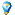###Gönderen Konu: Tübitak Lise Takım Seçme 2016 Soru 7  (Okunma sayısı 1550 defa)

####Eray

• G.O Genel Moderator
• G.O Efsane Üye
•• İleti: 352
• Karma: +8/-0##### Tübitak Lise Takım Seçme 2016 Soru 7
« : Nisan 04, 2016, 07:57:48 ös »
$A_1,A_2,\dots A_k$ $\{1,2,\dots, 2016\}$ kümesinin farklı alt kümeleri olmak üzere her $1\le i<j\le2016$ için $A_i\cap A_j$ bir aritmetik dizi oluşturuyorsa, $k$ nın alabileceği en büyük değer nedir?

(Azer Kerimov)
« Son Düzenleme: Nisan 23, 2016, 12:54:45 ös Gönderen: geo »
Matematik bilimlerin sultanıdır
-Carl Friedrich Gauss

####MATSEVER 27

• Geo-Maniac
••• İleti: 738
• Karma: +10/-6##### Ynt: Tübitak Lise Takım Seçme 2016 Soru 7
« Yanıtla #1 : Nisan 21, 2016, 07:30:20 ös »
Tayvan 2000 Soru 5. Let $n$ be a positive integer and $A=\{ 1,2,\ldots ,n\}$. A subset of $A$ is said to be connected if it consists of one element or several consecutive elements. Determine the maximum $k$ for which there exist $k$ distinct subsets of $A$ such that the intersection of any two of them is connected.

Evet haklısınız. Bu soru ancak bir örnek teşkil edebilir.
« Son Düzenleme: Nisan 23, 2016, 12:55:02 ös Gönderen: geo »
Vatan uğrunda ölen varsa vatandır.

####Eray

• G.O Genel Moderator
• G.O Efsane Üye
•• İleti: 352
• Karma: +8/-0##### Ynt: Tübitak Lise Takım Seçme 2016 Soru 7
« Yanıtla #2 : Nisan 21, 2016, 07:59:52 ös »
Tayvan 2000 Soru 5. Let $n$ be a positive integer and $A=\{ 1,2,\ldots ,n\}$. A subset of $A$ is said to be connected if it consists of one element or several consecutive elements. Determine the maximum $k$ for which there exist $k$ distinct subsets of $A$ such that the intersection of any two of them is connected.

Bu soruyla Türkiye TST 2016 P8 arasında bir fark var mı? Varsa da ben anlayamamış olabilirim. $n$ için yanıt $\binom{n}{2} + \binom{n}{1} + \binom{n}{0}$

Paylaştığınız soruda consecutive derken ardışık olmasını kastediyor. Yani ortak farkı 1 olan aritmetik dizi. Bu Türkiye sorusunda ise her türlü aritmetik dizi olabilir.
« Son Düzenleme: Nisan 23, 2016, 12:55:05 ös Gönderen: geo »
Matematik bilimlerin sultanıdır
-Carl Friedrich Gauss